# Generating Primitive Shapes in C++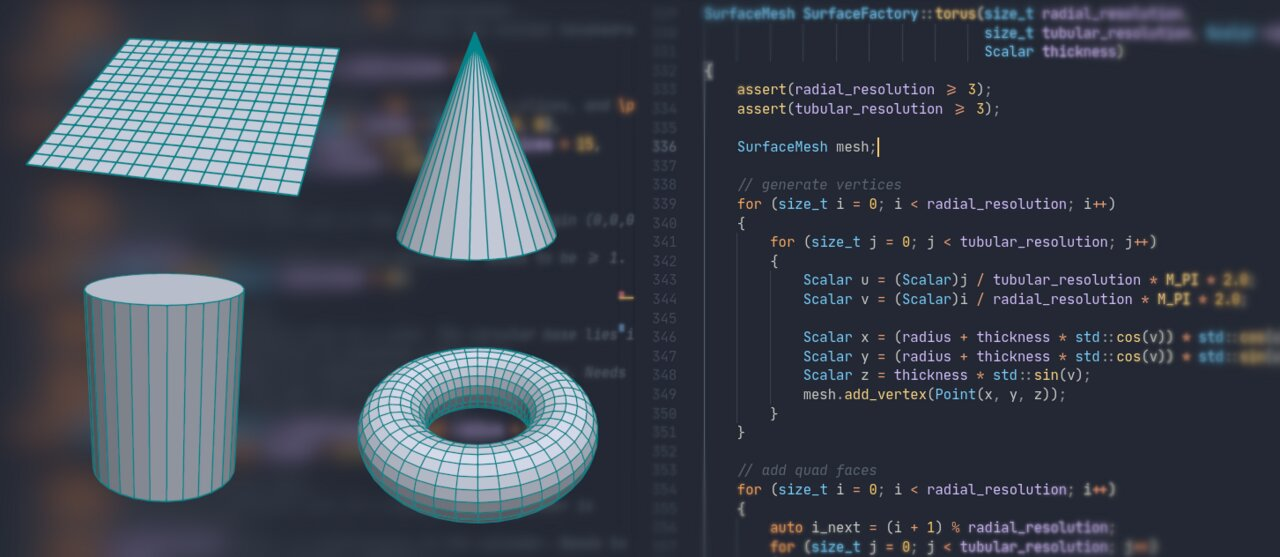This is the last part of my brief series on procedural mesh generation of simple shapes in C++. The two previous articles covered generating Platonic solids and different types of spheres. I recommend reading the other articles first, especially if you want to try out the code yourself.

This part covers the following primitives:

• Plane
• Cone
• Cylinder
• Torus

As with the previous articles, the code is in C++, using the PMP library, and written with a focus on simplicity. I only expose a minimal set of options and don’t really optimize for performance. However, you can easily extend the code to control additional properties such as position, size, and orientation.

## The Plane

Let’s start with the simplest shape, the plane. In the most trivial case, this is just single quad with four vertices lying in the plane:

``````SurfaceMesh plane()
{
SurfaceMesh mesh;

// add vertices in x-y plane

return mesh;
}
``````

While the above is fine for basic applications, you typically want to be able to refine the mesh into smaller elements, such as shown in the sequence below.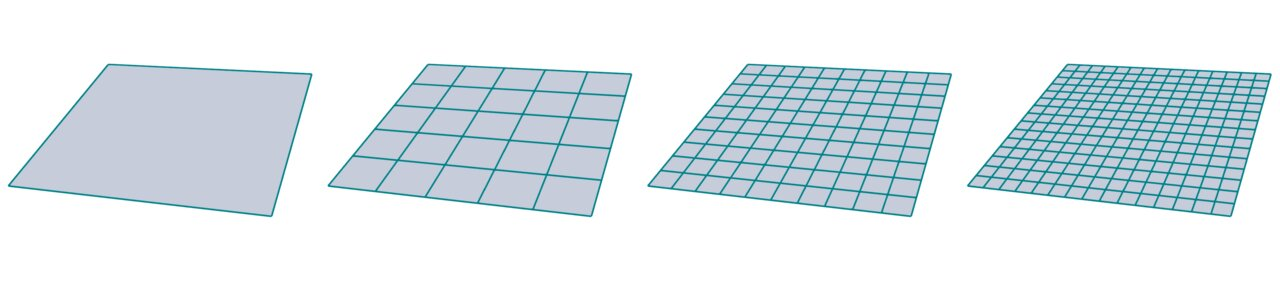Generating such a regular grid isn’t that much more complicated. All you need is two nested `for` loops to generate vertices and faces. For the sake of simplicity, I fix the resolution to be the same in each direction.

``````SurfaceMesh plane(size_t resolution)
{
SurfaceMesh mesh;

// generate vertices
Point p(0, 0, 0);
for (size_t i = 0; i < resolution + 1; i++) {
for (size_t j = 0; j < resolution + 1; j++) {
p += 1.0 / resolution;
}
p = 0;
p += 1.0 / resolution;
}

// generate faces
for (size_t i = 0; i < resolution; i++) {
for (size_t j = 0; j < resolution; j++) {
auto v0 = Vertex(j + i * (resolution + 1));
auto v1 = Vertex(v0.idx() + resolution + 1);
auto v2 = Vertex(v0.idx() + resolution + 2);
auto v3 = Vertex(v0.idx() + 1);
}
}

return mesh;
}
``````

If you’re curious to experiment with the code, go ahead and de-couple the resolution for the different plane directions, or add options for position, size, and orientation.

## The Cone

Next, let’s have a look at a slightly more complex shape, the cone. A cone basically consists of a circular base connected to a single tip or apex.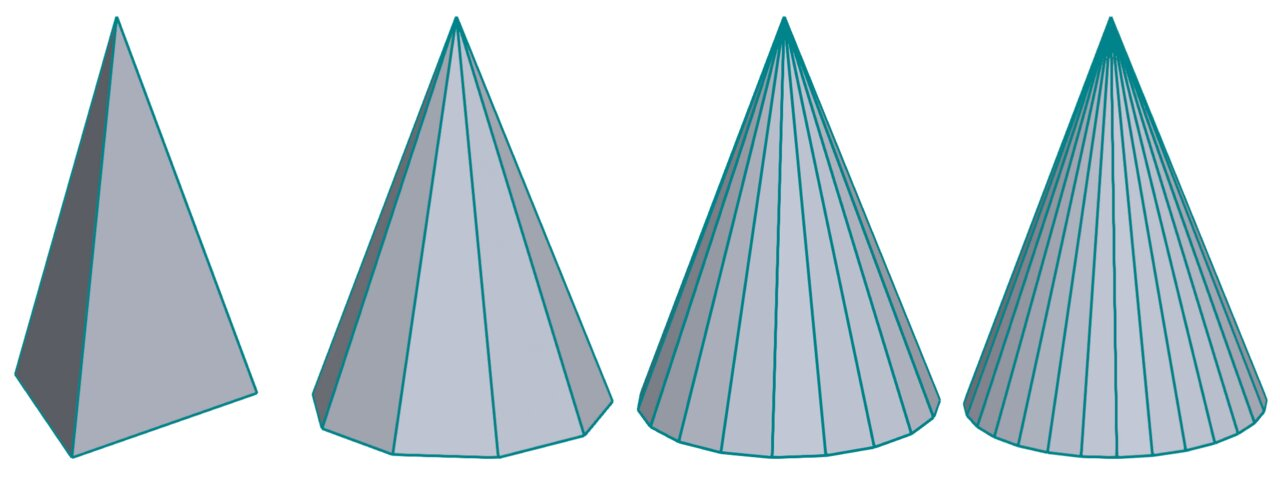The coarsest approximation to a cone is a tetrahedron (the leftmost image above), which you already saw in the tutorial about Platonic solids.

The code for generating a cone follows the same pattern that you are familiar with by now: Generate vertices first and then connect the faces.

For generating vertices, I sample a circle in the x-y plane, controlled by parameters for `resolution` and `radius`. I offset the tip vertex in z direction by `height` and add it last.

Adding faces is as simple as connecting two consecutive vertices from the base circle to the tip vertex. Finally, I add a single polygonal face to close the bottom of the cone.

``````SurfaceMesh cone(size_t resolution,
Scalar height)
{
SurfaceMesh mesh;

// add vertices subdividing a circle
std::vector<Vertex> base_vertices;
for (size_t i = 0; i < resolution; i++) {
Scalar ratio = static_cast<Scalar>(i) / (resolution);
Scalar r = ratio * (M_PI * 2.0);
Scalar x = std::cos(r) * radius;
Scalar y = std::sin(r) * radius;
auto v = mesh.add_vertex(Point(x, y, 0.0));
base_vertices.push_back(v);
}

// add the tip of the cone
auto v0 = mesh.add_vertex(Point(0.0, 0.0, height));

// generate triangular faces
for (size_t i = 0; i < resolution; i++) {
auto ii = (i + 1) % resolution;
}

// reverse order for consistent face orientation
std::reverse(base_vertices.begin(), base_vertices.end());

return mesh;
}
``````

## The Cylinder

Next, let’s have a look at cylinders which are barely more complicated than cones. To be more precise, I consider discrete approximations to a cylinder which are prisms, i.e., n-sided polygonal base faces parallel to each other connected by n other quadrilateral faces joining the two base faces.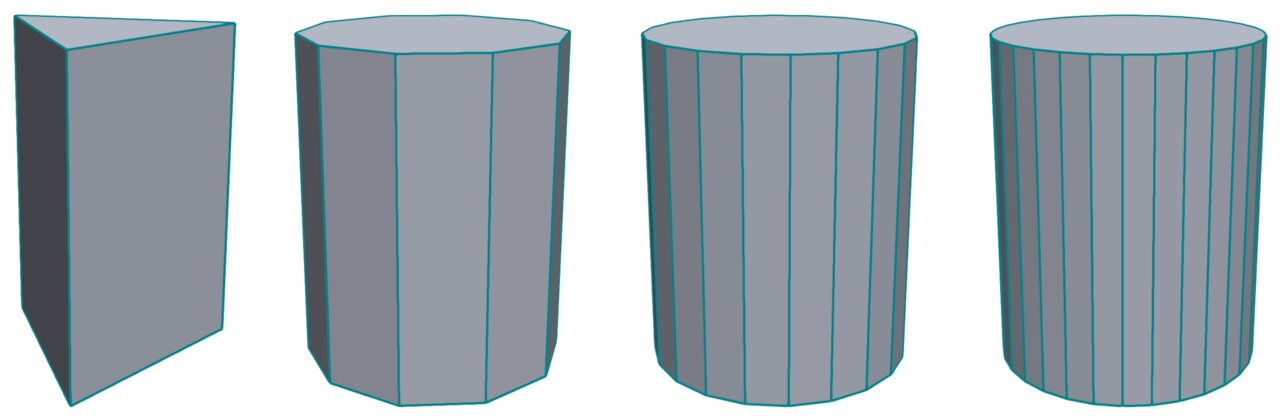Generating a cylinder is very similar to generating a cone as described above. The major difference is that I sample two different base circles and generate quadrilateral faces connecting pairs of vertices from those two circles.

``````SurfaceMesh cylinder(size_t resolution,
Scalar height)
{
SurfaceMesh mesh;

// generate vertices
std::vector<Vertex> bottom_vertices;
std::vector<Vertex> top_vertices;
for (size_t i = 0; i < resolution; i++) {
Scalar ratio = static_cast<Scalar>(i) / (resolution);
Scalar r = ratio * (M_PI * 2.0);
Scalar x = std::cos(r) * radius;
Scalar y = std::sin(r) * radius;
Vertex v = mesh.add_vertex(Point(x, y, 0.0));
bottom_vertices.push_back(v);
top_vertices.push_back(v);
}

// add faces around the cylinder
for (size_t i = 0; i < resolution; i++) {
auto ii = i * 2;
auto jj = (ii + 2) % (resolution * 2);
auto kk = (ii + 3) % (resolution * 2);
auto ll = ii + 1;
}

// reverse order for consistent face orientation
std::reverse(bottom_vertices.begin(), bottom_vertices.end());

return mesh;
}
``````

## The Torus

The final shape I’ll describe in this tutorial is the well-known torus or doughnut shape. A torus is generated by revolving a circle in three-dimensional space about an axis that is coplanar with a circle.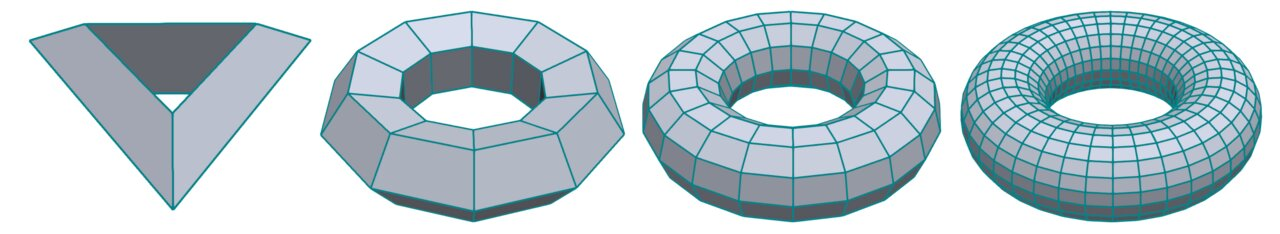The code pattern remains the same: Vertex generation comes first, then add the corresponding faces. The indexing of the faces gets a bit more tricky due to the nested `for` loops, but that’s basically about it. I use some intermediate variables to make the code clearer, hope this helps.

``````SurfaceMesh torus(size_t radial_resolution,
size_t tubular_resolution,
Scalar thickness)
{
SurfaceMesh mesh;

// generate vertices
for (size_t i = 0; i < radial_resolution; i++) {
for (size_t j = 0; j < tubular_resolution; j++) {
Scalar u = (Scalar)j / tubular_resolution * M_PI * 2.0;
Scalar v = (Scalar)i / radial_resolution * M_PI * 2.0;
Scalar x = (radius + thickness * std::cos(v)) * std::cos(u);
Scalar y = (radius + thickness * std::cos(v)) * std::sin(u);
Scalar z = thickness * std::sin(v);
}
}

for (size_t i = 0; i < radial_resolution; i++) {
auto i_next = (i + 1) % radial_resolution;
for (size_t j = 0; j < tubular_resolution; j++) {
auto j_next = (j + 1) % tubular_resolution;
auto i0 = i * tubular_resolution + j;
auto i1 = i * tubular_resolution + j_next;
auto i2 = i_next * tubular_resolution + j_next;
auto i3 = i_next * tubular_resolution + j;
}
}

return mesh;
}
``````

## Closing Remarks

This finishes my brief series on procedural mesh generation of simple shapes. Together with part I and part II this should provide you with a solid basis for creating simple shapes in a modeling application or to test an algorithm against shapes with known properties.

The complete code is available as well. In order to try it out, just copy the file to `src/MyViewer.cpp` in the PMP project template, build the project, and run the `./myviewer` executable.

Comments or questions? Simply drop me a mail.